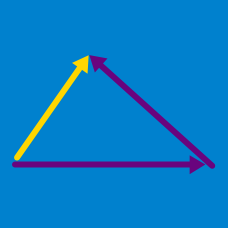Geometry

# Properties of a Vector: Level 1 Challenges

A helicopter takes off from a military base to pick up three soldiers. Initially it flies 10 miles east to pick up the first, then it flies 20 miles north to pick up the second, and lastly flies 10 miles west to pick up the third. How far and in what direction is the helicopter from the base?

Suppose two vectors $\vec{u}$ and $\vec{v}$ have the same magnitude but have opposite directions. What is $\vec{u} + \vec{v}$?

A bug flies from the point $P(3, 4, 5)$ to the point $Q(-1, 7, 4)$. However, the bug is very indecisive and decides to fly three times as far in the opposite direction after landing at $Q$. Where will the bug land after this trip?

Can $\langle 6, 8 \rangle$ be expressed as the sum of nine unit vectors?

Which vector represents the unit vector in the direction of $\langle 3, -6, 2\rangle$?

×